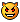# Giving a number a differnet value?

## Recommended Posts

• Moderators

Hey all, I thought I saw a few days ago a similar post, but I can't seem to find it.

I'm trying to get a variable to translate to a different value.

\$var = "1234567890"

Trying to give each number a specific value like this.

1 = "h"

2 = "I"

3 = "3"

4 = "R"

5 = "J"

6 = "6"

7 = "b"

8 = "Z"

9 = "9"

0 = "00"

So if you got \$var = "1234567890" and translated it to = "hI3RJ6bZ900" or if you had \$var = 22315994 it would be "II3hJ99R".

I'm sure it's using the arrays, but my homework is lacking today (besides I suck at them).

Any help is appreciated.

Common sense plays a role in the basics of understanding AutoIt... If you're lacking in that, do us all a favor, and step away from the computer.

##### Share on other sites
• Developers

```Dim \$Codes
\$Codes = "00"
\$Codes = "h"
\$Codes = "i"
\$Codes = "3"
\$Codes = "R"
\$Codes = "j"
\$Codes = "6"
\$Codes = "b"
\$Codes = "Z"
\$Codes = "9"
;
\$var = "1234567890"
\$Out_Var = ""
For \$x = 1 to StringLen(\$var)
\$i = number(StringMid(\$var,\$x,1))
\$Out_Var = \$Out_Var & \$Codes[\$i]
Next
MsgBox(0,'test',\$Out_Var)```

Live for the present,
Dream of the future,
Learn from the past.##### Share on other sites
• Moderators

Thanks and that was quick: Ask for help get the "answer". I'll study this so that I can understand exactly what you did!!Thanks again JdeBEdit: Wish I could spell!!

Edited by ronsrules

Common sense plays a role in the basics of understanding AutoIt... If you're lacking in that, do us all a favor, and step away from the computer.

## Create an account

Register a new account

• ### Recently Browsing   0 members

×

• Wiki

• Back

• #### Beta

• Git
• FAQ
• Our Picks
×
• Create New...